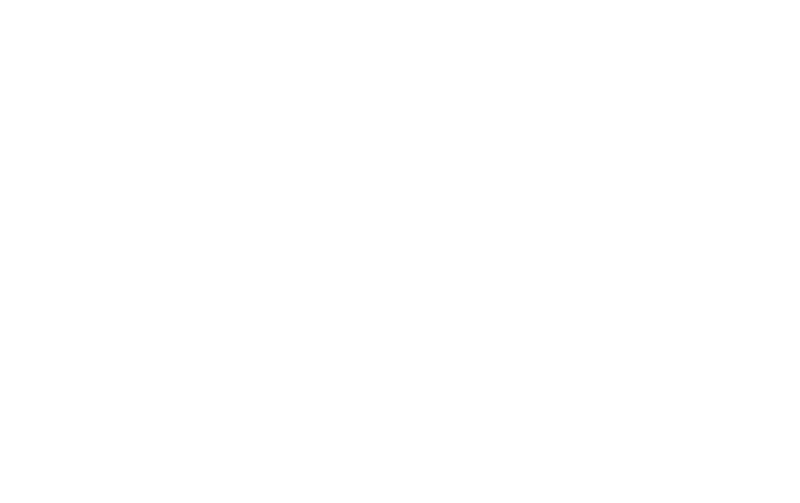GitHub Repo: https://github.com/ianhi/AC29…

### 介绍

1. 手动标记自己的图像

1. 通过迁移学习建立有效的分割模型

1. 可视化模型及其结果

1. 以Docker映像形式共享你的项目

### 1.标签

Labelme： https://github.com/wkentaro/l…
ImageJ： https://imagej.net/Welcome
GIMP： https://www.gimp.org/

1. 用鼠标选择图像的一部分

1. 轻松在图像之间切换并选择要标记的类别

LassoSelector： https://matplotlib.org/3.1.1/…

LassoSelector会处理一条线以显示你所选择的内容，但是我们需要一些自定义代码来将掩膜绘制为覆盖层：

```import numpy as np
import matplotlib.pyplot as plt
from matplotlib.widgets import LassoSelector
from matplotlib.path import Path
class image_lasso_selector:
"""
img must have shape (X, Y, 3)
"""
self.img = img
plt.ioff() # see https://github.com/matplotlib/matplotlib/issues/17013
self.fig = plt.figure(figsize=figsize)
self.ax = self.fig.gca()
self.displayed = self.ax.imshow(img)
plt.ion()

lineprops = {'color': 'black', 'linewidth': 1, 'alpha': 0.8}
self.lasso = LassoSelector(self.ax, self.onselect,lineprops=lineprops, useblit=False)
self.lasso.set_visible(True)

pix_x = np.arange(self.img.shape)
pix_y = np.arange(self.img.shape)
xv, yv = np.meshgrid(pix_y,pix_x)
self.pix = np.vstack( (xv.flatten(), yv.flatten()) ).T

def onselect(self, verts):
self.verts = verts
p = Path(verts)

array = self.displayed.get_array().data
# https://en.wikipedia.org/wiki/Alpha_compositing#Straight_versus_premultiplied
self.displayed.set_data(array)
self.fig.canvas.draw_idle()

def _ipython_display_(self):
display(self.fig.canvas)```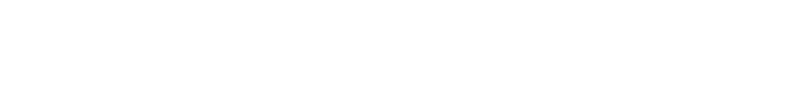https://youtu.be/aYb17GueVcU

### 2.模型训练

#### 模型

U-net是一个卷积神经网络，最初设计用于分割生物医学图像，但已成功用于许多其他类型的图像。它以现有的卷积网络为基础，可以在很少的训练图像的情况下更好地工作，并进行更精确的分割。这是一个最新模型，使用segmentation_models库也很容易实现。

segmentation_models库： https://github.com/qubvel/seg…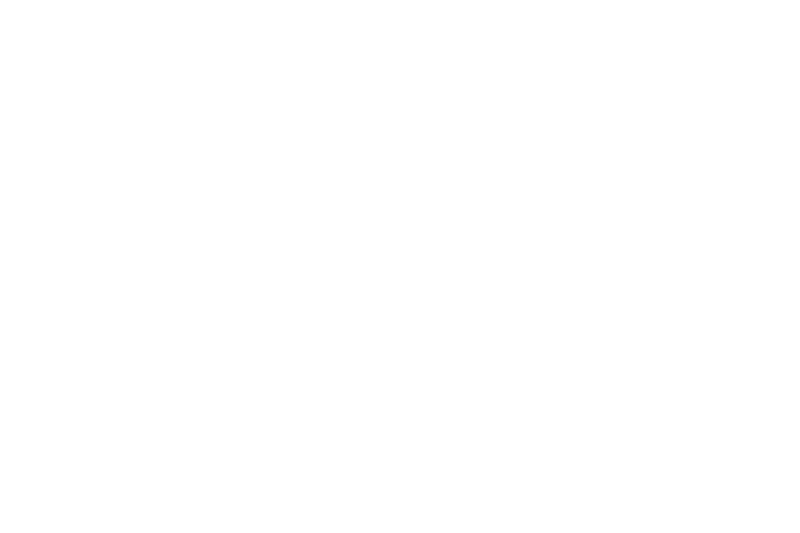U-net的独特之处在于它通过交叉连接将编码器和解码器结合在一起（上图中的灰色箭头）。这些跳跃连接从下采样路径中的相同大小的部分跨到上采样路径。这样可以提高你对上采样时输入到模型中的原始像素的了解，这已被证明可以提高分割任务的性能。

#### 数据扩充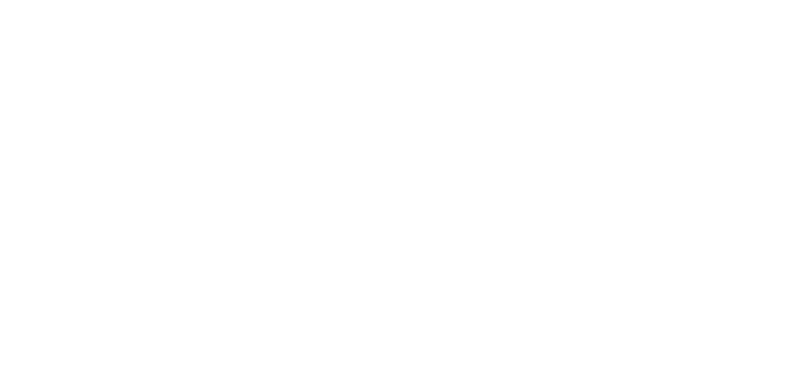```import albumentations as A
import numpy as np
from tensorflow.keras.preprocessing.image import ImageDataGenerator
TARGET_SIZE = (224,224)
BATCH_SIZE = 6
def create_augmentation_pipeline():
augmentation_pipeline = A.Compose(
[
A.HorizontalFlip(p = 0.5), # Apply horizontal flip to 50% of images
A.OneOf(
[
# Apply one of transforms to 50% of images
A.RandomContrast(), # Apply random contrast
A.RandomGamma(), # Apply random gamma
A.RandomBrightness(), # Apply random brightness
],
p = 0.5
),
A.OneOf(
[
# Apply one of transforms to 50% images
A.ElasticTransform(
alpha = 120,
sigma = 120 * 0.05,
alpha_affine = 120 * 0.03
),
A.GridDistortion()
],
p = 0.5
)
],
p = 0.9 # In 10% of cases keep same image because that's interesting also
)
return augmentation_pipeline
def create_datagenerator(PATH):
options = {'horizontal_flip': True, 'vertical_flip': True}
image_datagen = ImageDataGenerator(rescale=1./255, **options)
val_datagen = ImageDataGenerator(rescale=1./255)

# Create custom zip and custom batch_size
def combine_generator(gen1, gen2, batch_size=6,training=True):
while True:
image_batch, label_batch = next(gen1), np.expand_dims(next(gen2)[:,:,0],axis=-1)
image_batch, label_batch = np.expand_dims(image_batch,axis=0), np.expand_dims(label_batch,axis=0)
for i in range(batch_size - 1):
image_i,label_i = next(gen1), np.expand_dims(next(gen2)[:,:,0],axis=-1)

if training == True:
aug_pipeline = create_augmentation_pipeline()
image_i, label_i = np.expand_dims(image_i,axis=0),np.expand_dims(label_i,axis=0)
image_batch = np.concatenate([image_batch,image_i],axis=0)
label_batch = np.concatenate([label_batch,label_i],axis=0)

yield((image_batch,label_batch))

seed = np.random.randint(0,1e5)
train_image_generator = image_datagen.flow_from_directory(PATH+'train_imgs', seed=seed, target_size=TARGET_SIZE, class_mode=None, batch_size=BATCH_SIZE)

val_image_generator = val_datagen.flow_from_directory(PATH+'val_imgs', seed=seed, target_size=TARGET_SIZE, class_mode=None, batch_size=BATCH_SIZE)

return train_generator, val_generator```

#### 整理

https://github.com/ianhi/AC29…

https://vimeo.com/419423808### 3.可视化

GitHub存储库： https://github.com/anktplwl91…

#### 中间层激活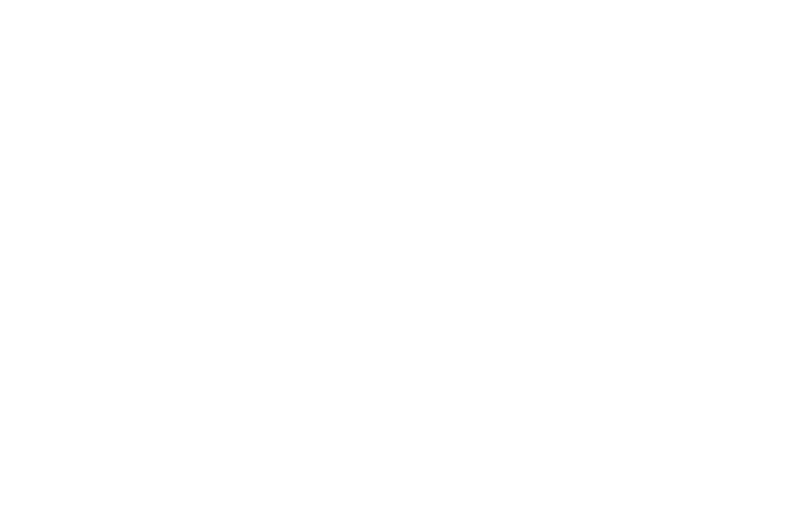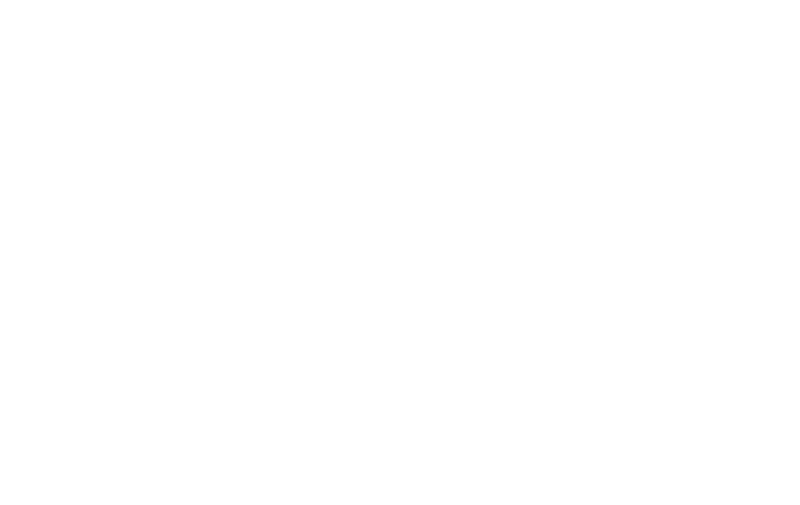#### 类激活的热图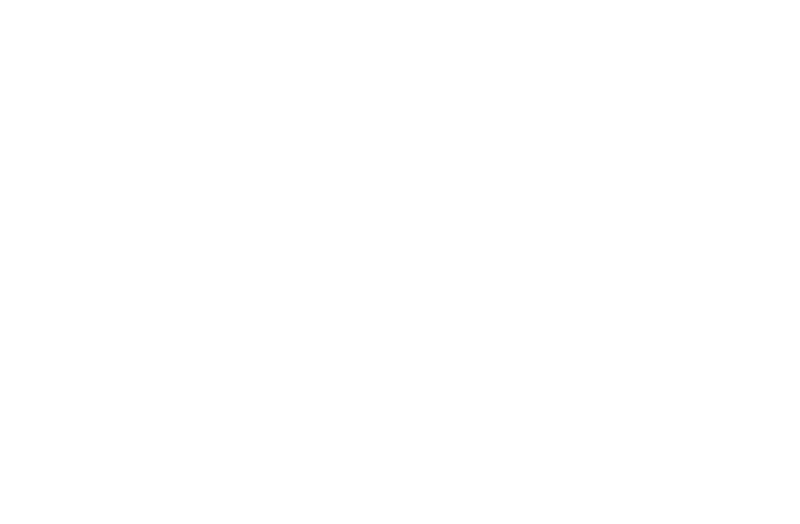### 4.制作和共享Docker映像

```sudo docker run -p 8888:8888 ianhuntisaak/ac295-final-project:v3 \
-e JUPYTER_LAB_ENABLE=yes```

### 结论与未来工作

1. 通过使用html5画布构建自定义Jupyter小部件来改善套索工具，以减少手动分割时的滞后

1. 探索新的损失函数和模型作为迁移学习的基础

1. 使解释可视化更加容易，并向用户建议改善结果的方法